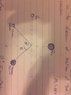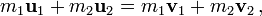# Momentum (Collision @ Angle)

• McKeavey
In summary, the problem involves a collision between a neutron and a helium nucleus, with given velocities and masses. The goal is to find the angle and velocity of the helium nucleus after the collision. To solve this problem, the basic principle of conservation of momentum is applied, and the given velocities are analyzed in components. This can be solved by setting up equations for conservation of momentum in both the x and y directions.

## Homework Statement

A neutron with velocity 5E5 m/s and a mass of 1.66E-27 collides with a helium nucleus, mass of 4(1.66E-27) with a velocity of 3.7E5 m/s as shown. If the speed of the neutron after collision is 5E5 m/s and it is moving in the direction of motion of the nucleus after collision, find theta, and velocity of Helium (Prime).
Diagram shown in attachment.
*also the angle 39 + theta does not equal 90 degrees

P = mv

## The Attempt at a Solution

My teacher assigned it to us and expects us to solve it somehow..
Please if someone could lead my through the steps of solving it..It'd be greatly appreciated.

Don't expect me to know anything..

#### Attachments

•456.jpg
21.1 KB · Views: 373
You apply the same basic principles of conservation of momentum, only now, you have to analyze it in components.

Which I do not know because I never learned it hah :/
I said I don't know anything about momentum :/

If you've done 2D kinematics, it should be really simple. Just remember the formula for conservation of momentum:where u1 and u2 are the velocities before collision, and v1 and v2 are the velocities after collision.

Split each velocity vector up into components and solve for what needs to be solved. So you should have the same equation (^) for both components except one will be analyzing the velocity vectors in the x -direction and one in the y-direction.